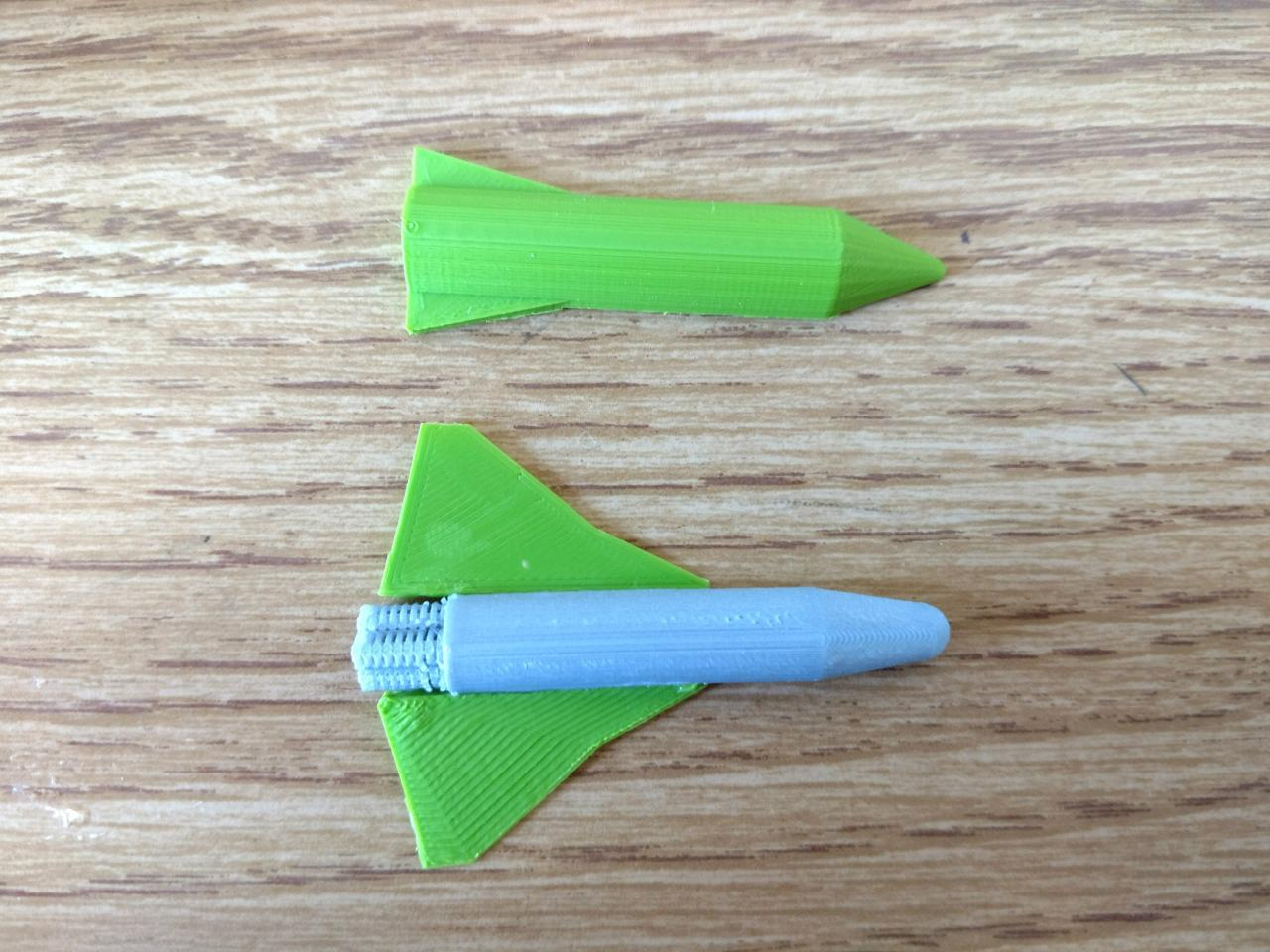# Rocket Ship

For OpenSCAD I found very quickly that I suck at it. I am definitely not a coder!

In frustration with all the little details I decided to focus and make small things and then increasing their difficulty. I started with a cone and cylinder and finished with making 2 polyhedrons. The latter 2 were very frustrating as I had to not only type in points but then specify how each point would connect to the next. I quickly say how cool this could be but considering all I wanted was a triangular prism, I found it annoying and unnecessary.

```///// objects ///top/// translate([0,0,15]) cylinder(h=15, r1=10, r2=0, center=true); ///base/// translate([0,0,-15]) cylinder(h=45, r=10, center=true); ///side one/// translate([0,0,-37.5]) polyhedron( points=[ [10,-2,0], [10,2,0], [15,-2,0], [15,2,0], [10,-2,20], [10,2,20] ], faces=[ [0,1,3,2], [5,4,2,3], [0,4,5,1], [0,2,4], [5,3,1] ] ); ///side two/// translate([0,0,-37.5]) polyhedron( points=[ [-10,2,0], [-10,-2,0], [-15,2,0], [-15,-2,0], [-10,2,20], [-10,-2,20] ], faces=[ [0,1,3,2], [5,4,2,3], [0,4,5,1], [0,2,4], [5,3,1] ] );```

The side one and side two are my triangular prisms on either side of my cylinder. It took my a couple hours and a lot of frustration to finally figure the first one out. Once that was done I just flipped it by changing a few points into negatives which resulted in side two!

One  challenge I had was remembering which point was which, so I would get this weird triangle in my prism because I would connect sides incorrectly!I finally wrote down everything to ensure it was correct! Then it was much easier to figure out what I wanted. Ultimately that was my main issue (and still is) with code. I have to draw what I want on a piece of paper to visualize everything correctly, otherwise I can’t figure out where I am going wrong! It makes everything that much more tedious but it is work it in the end!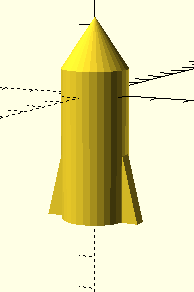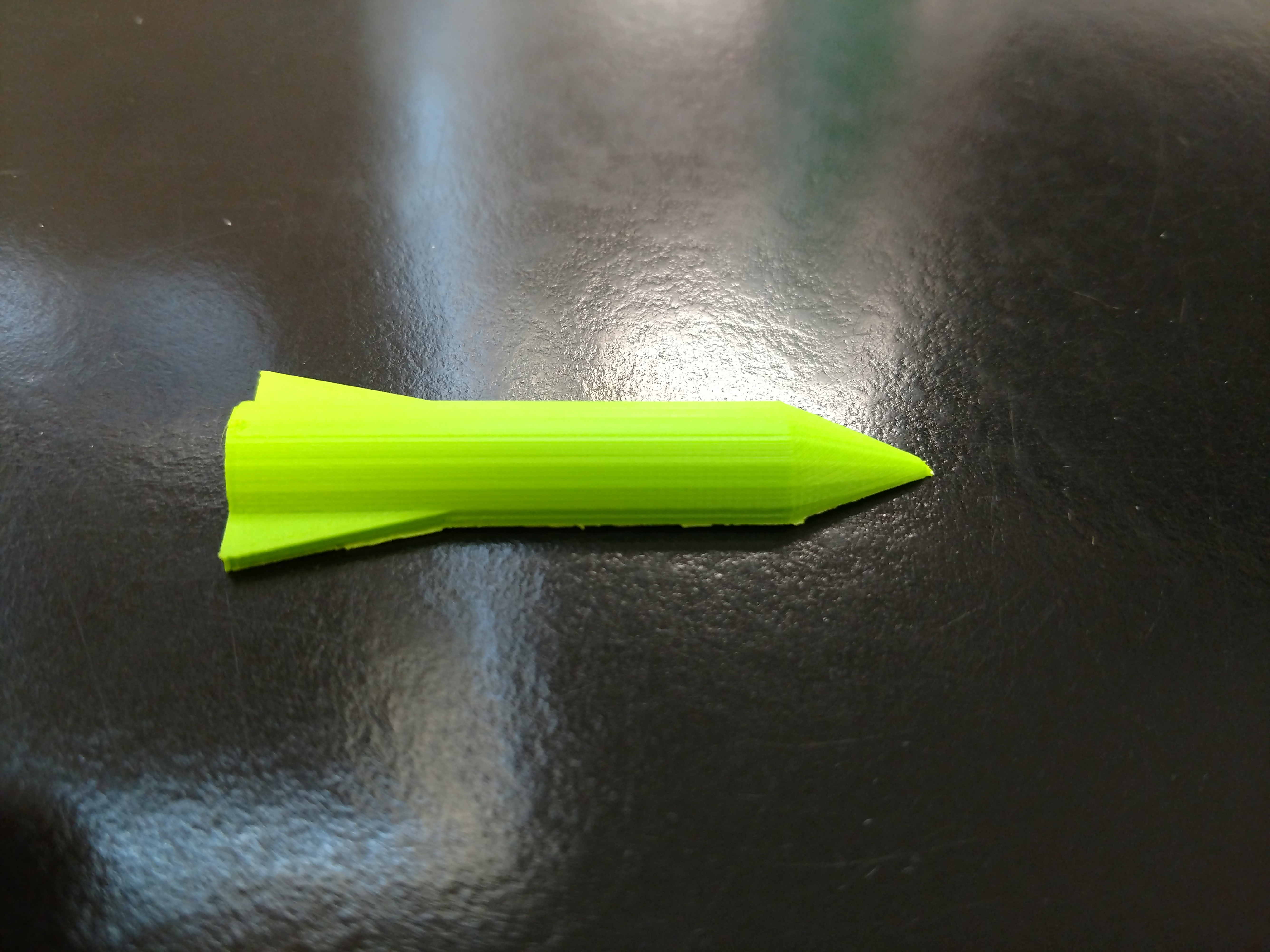## Final Rocket

I decided that for my final project I wanted the rocket to look more aerodynamic. I added rockets on the bottom and slimmed down the main part. I also worked on the wings to look slimmer, and overall more like wings a rocket would have.

```///Main Part///
///top///
difference() {
difference() {
translate([0,0,8])
cylinder(h=12, r1=5, r2=3, center=true, \$fn=200);
translate([0,5,8])
cube( size= [12,6,17], center=true);
}
}
;
difference() {
translate([0,0,13.21])
sphere( r=3.1, center=true, \$fn=200);
translate([0,5,])
cube( size= [12,6,12], center=true);
}
///base///
difference() {
translate([0,0,-17])
cylinder(h=38, r=5, center=true, \$fn=200);
translate([0,5,-15])
cube( size= [12,6,50], center=true);
}

///Wing One///
polyhedron(
points=[ [4.6,-.5,-45], [4.6,2.005,-45], [4.4,2.005,-5], [11.8,2.005,-20], [24.8,2.005,-35], [24.8,2.005,-40] ],
faces=[ [0,1,2], [0,2,3,4,5], [1,2,3,4,5], [0,1,5] ]
);
////Wing Two////
polyhedron(
points=[ [-4.6,-.5,-45], [-4.6,2.005,-45], [-4.4,2.005,-5], [-11.8,2.005,-20], [-24.8,2.005,-35], [-24.8,2.005,-40] ],
faces=[ [0,1,2], [0,2,3,4,5], [1,2,3,4,5], [0,1,5] ]
);

///Rockets///

/// Rocket One///
difference() {
translate([2.5,0.1,-40])
cylinder(h=10, r=1.7, center=true, \$fn=200);
translate([2.5,0.1,-40])
cylinder(h=11, r=1.5, center=true, \$fn=200);
}
/// Rocket Two///
difference() {
translate([-2.5,.1,-40])
cylinder(h=10, r=1.7, center=true, \$fn=200);
translate([-2.5,.1,-40])
cylinder(h=11, r=1.5, center=true, \$fn=200);
}
/// Rocket Three///
difference() {
translate([0,-2.5,-40])
cylinder(h=10, r=1.7, center=true, \$fn=200);
translate([0,-2.5,-40])
cylinder(h=11, r=1.5, center=true, \$fn=200);
}
///Rocket Edits///
/////
///Rockets///
/// Rocket One///

/// Rocket Two///

/// Rocket Three///

///parameters///
a=-40;
b=.1;
c=2.5;
/////
difference () {
{

translate([c,b,a])
cylinder(h=10, r=2, center=true, \$fn=200);

}
//////////
/// parameters///
x=-30;
//// cuts///
{

translate([c,b,x-7])
cube( size=[4,4,.5], center=true);
translate([c,b,x-8])
cube( size=[4,4,.5], center=true);
translate([c,b,x-9])
cube( size=[4,4,.5], center=true);
translate([c,b,x-10])
cube( size=[4,4,.5], center=true);
translate([c,b,x-11])
cube( size=[4,4,.5], center=true);
translate([c,b,x-12])
cube( size=[4,4,.5], center=true);
translate([c,b,x-13])
cube( size=[4,4,.5], center=true);
translate([c,b,x-14])
cube( size=[4,4,.5], center=true);
translate([c,b,x-15])
cube( size=[4,4,.5], center=true);
translate([c,b,x-16])
cube( size=[4,4,.5], center=true);
translate([c,b,x-17])
cube( size=[4,4,.5], center=true);

}
}
///parameters///
a=-40;
b=.1;
c=2.5;
/////
difference () {
{

translate([-c,b,a])
cylinder(h=10, r=2, center=true, \$fn=200);

}
//////////
/// parameters///
x=-30;
//// cuts///
{

translate([-c,b,x-7])
cube( size=[4,4,.5], center=true);
translate([-c,b,x-8])
cube( size=[4,4,.5], center=true);
translate([-c,b,x-9])
cube( size=[4,4,.5], center=true);
translate([-c,b,x-10])
cube( size=[4,4,.5], center=true);
translate([-c,b,x-11])
cube( size=[4,4,.5], center=true);
translate([-c,b,x-12])
cube( size=[4,4,.5], center=true);
translate([-c,b,x-13])
cube( size=[4,4,.5], center=true);
translate([-c,b,x-14])
cube( size=[4,4,.5], center=true);
translate([-c,b,x-15])
cube( size=[4,4,.5], center=true);
translate([-c,b,x-16])
cube( size=[4,4,.5], center=true);
translate([-c,b,x-17])
cube( size=[4,4,.5], center=true);

}
}

///parameters///
a=-40;
d=-2.5;
e=0;
/////
difference () {
{

translate([e,d,a])
cylinder(h=10, r=2, center=true, \$fn=200);

}
//////////
/// parameters///
x=-30;
//// cuts///
{

translate([e,d,x-7])
cube( size=[4,4,.5], center=true);
translate([e,d,x-8])
cube( size=[4,4,.5], center=true);
translate([e,d,x-9])
cube( size=[4,4,.5], center=true);
translate([e,d,x-10])
cube( size=[4,4,.5], center=true);
translate([e,d,x-11])
cube( size=[4,4,.5], center=true);
translate([e,d,x-12])
cube( size=[4,4,.5], center=true);
translate([e,d,x-13])
cube( size=[4,4,.5], center=true);
translate([e,d,x-14])
cube( size=[4,4,.5], center=true);
translate([e,d,x-15])
cube( size=[4,4,.5], center=true);
translate([e,d,x-16])
cube( size=[4,4,.5], center=true);
translate([e,d,x-17])
cube( size=[4,4,.5], center=true);

}
}```

Altogether my code looked great, the rocket looked nice and it seemed as though it would print smoothly, but alas, code and I do not in fact get along.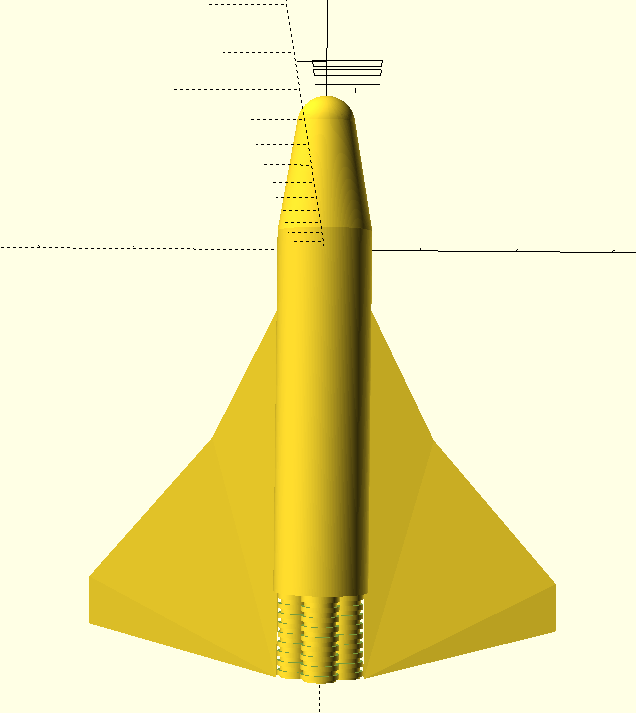When I tried to print for some reason Cura decided that my wing on the right (Wing Two) was inadequate. I tackled with the program for some time but no matter how I changed the code, Wing Two was unstable and didn’t want to look good on Cura. I decided to let it print and see how horrible it could possibly turn out.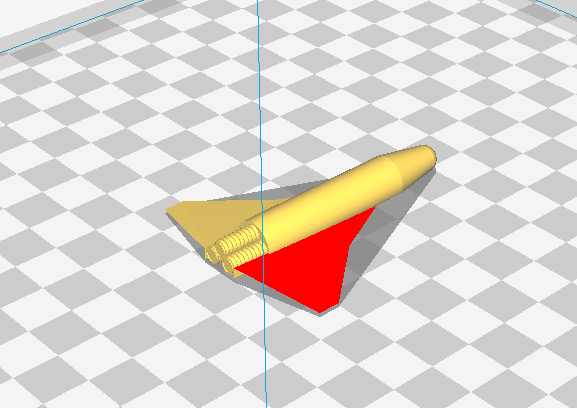Almost ironically, the wings didn’t print out at all! I still don’t understand why neither printed but I figured I would just have to go along with the punches since I couldn’t seem to change the code in a way Cura would be happy with.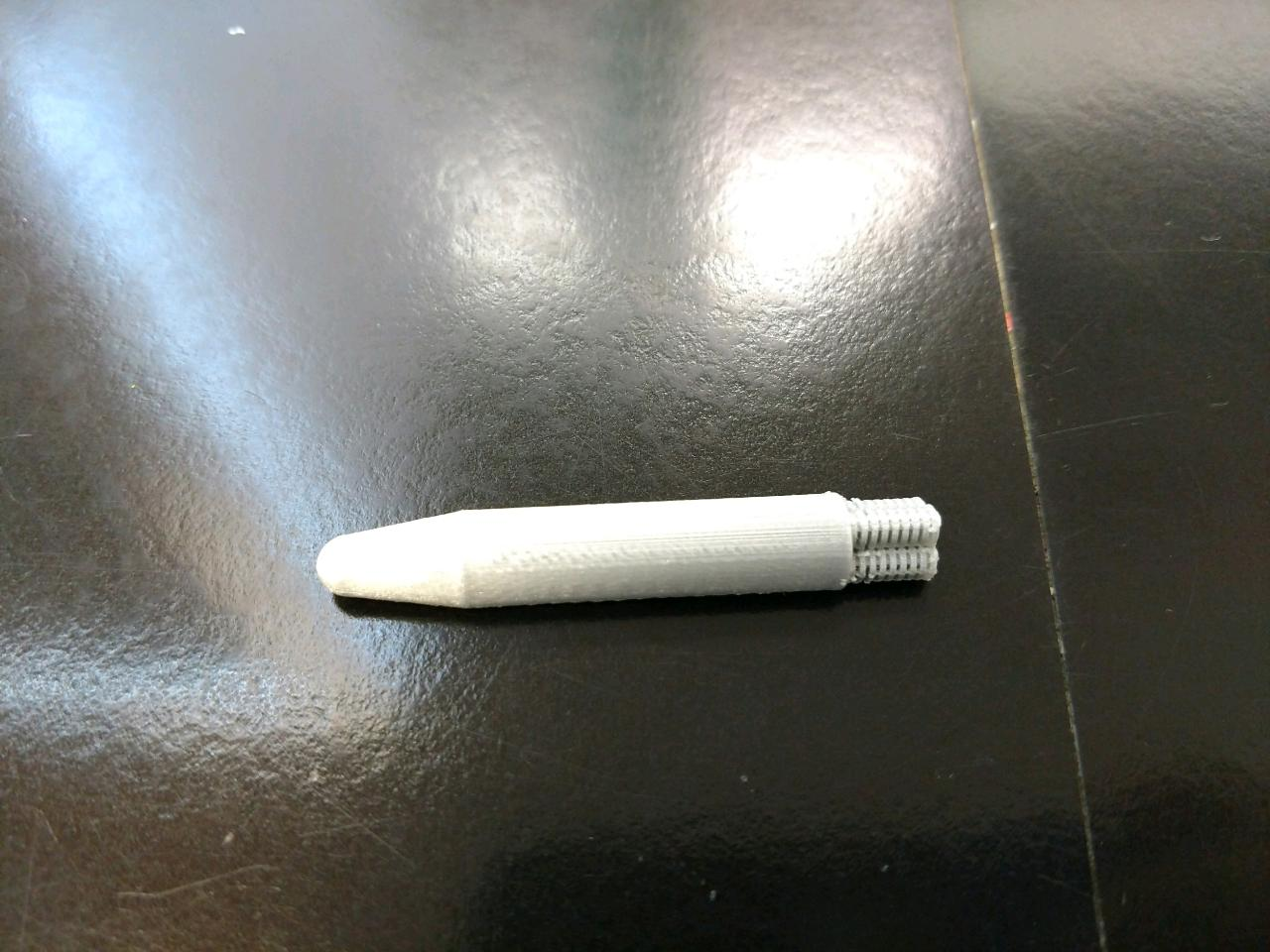With acceptance of failure to print the rocket at a whole, I decided I would just print each wing individually.

Once again, when I went to print out Wing Two was red. So I accepted code defeat and went foreword using only Wing One. It didn’t cause too much of an issue considering the code for both wings is exactly the same other than the x-values being negative.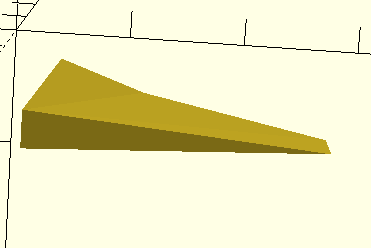The wings turned out perfectly! I flipped Wing One in Cura and had no issues. The only thing left was to connect the wings, which I had no issues with thanks to super glue.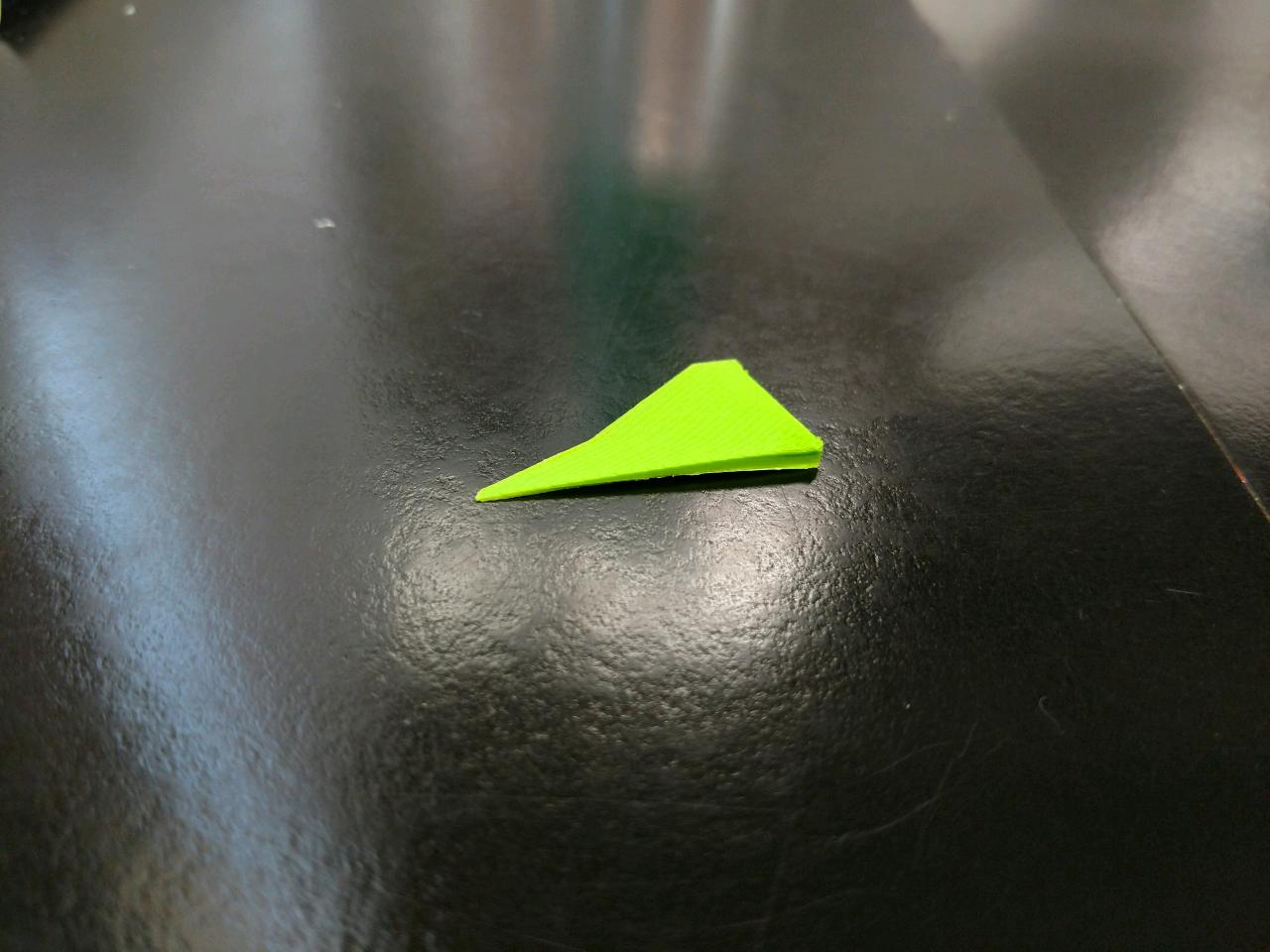It’s small and bicolored, which I actually like about it. It works as a small figurine.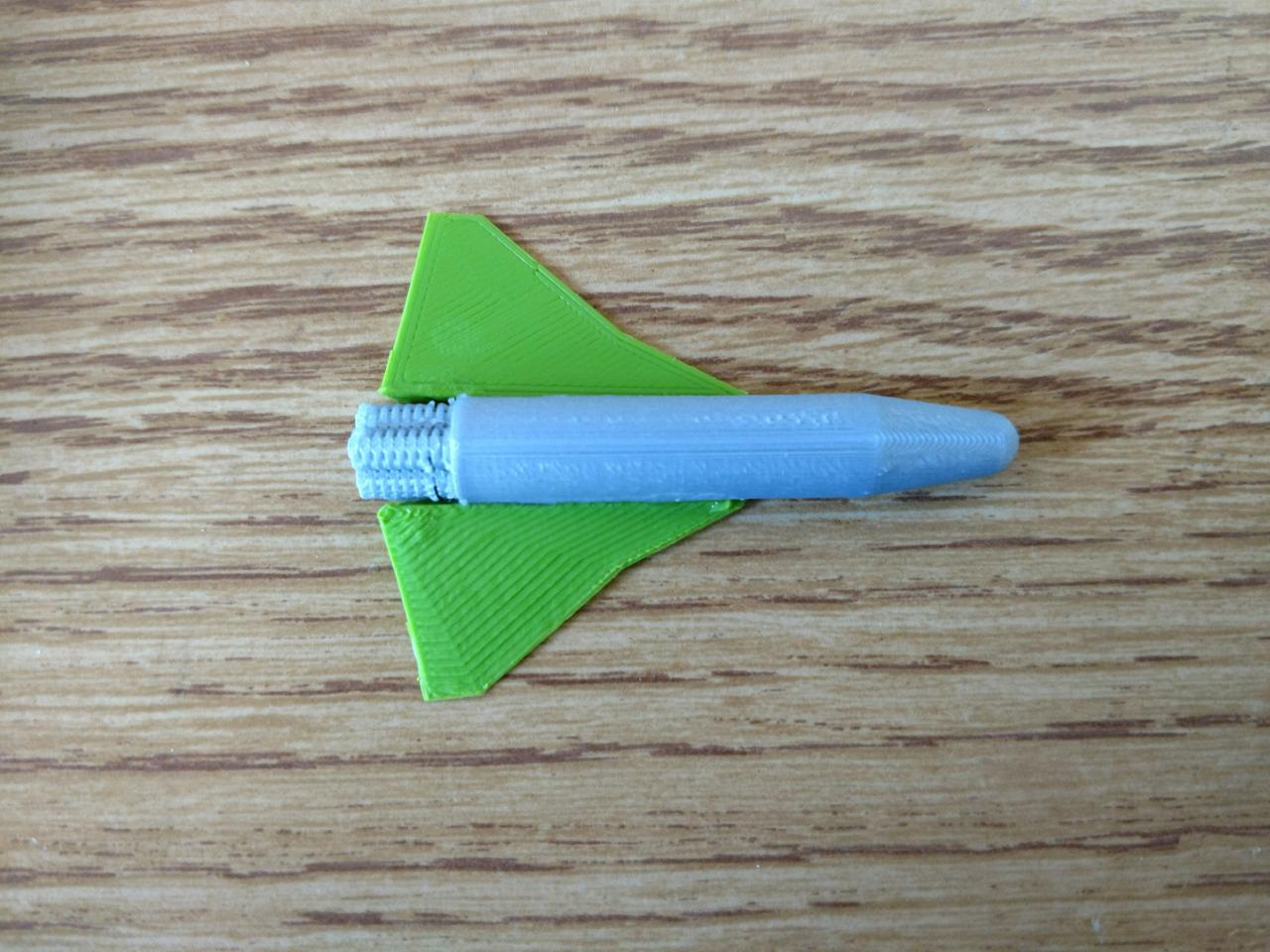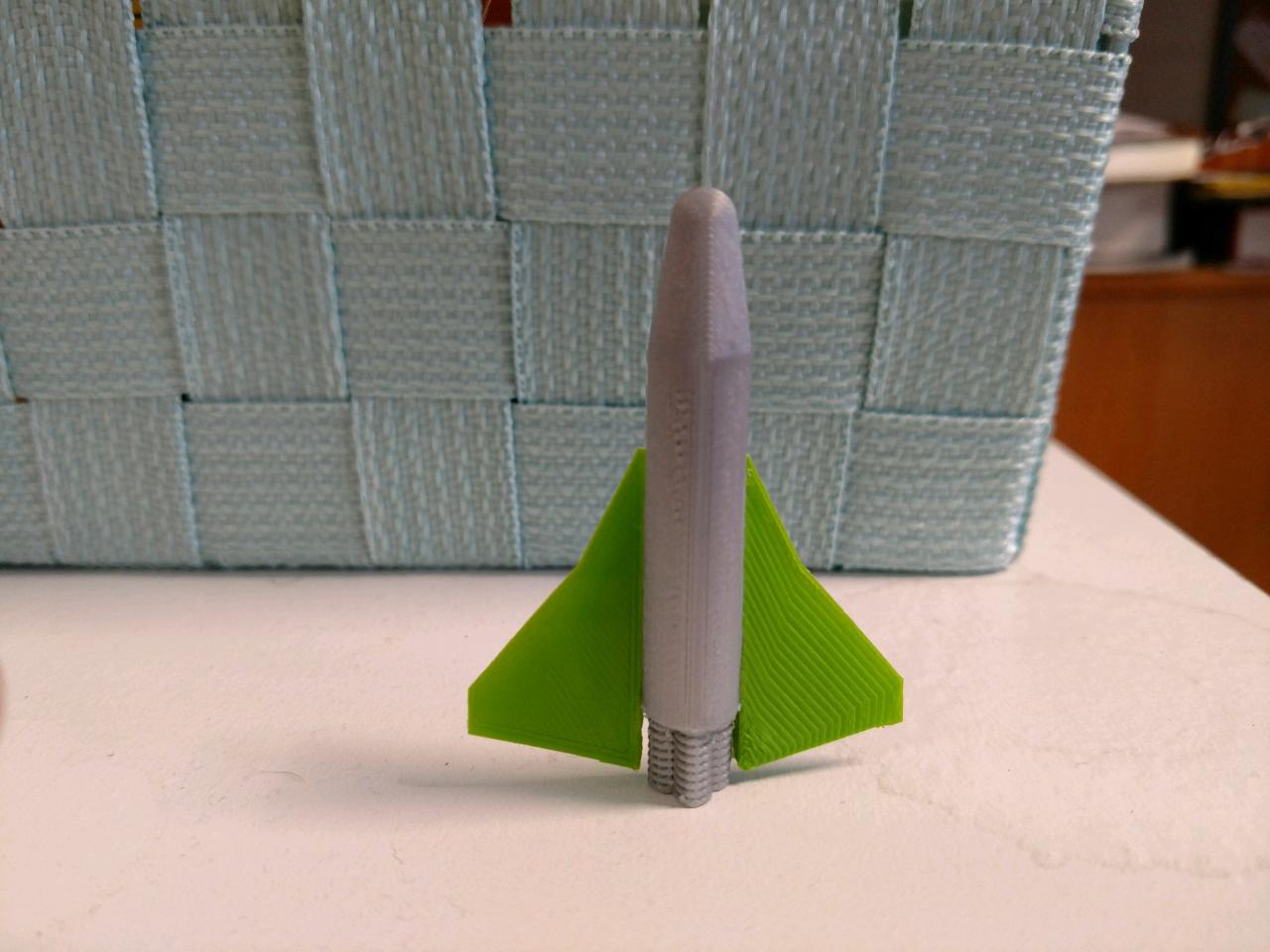Here is the former and latter next to each other! You can see the differences are pretty drastic. The Rocket turned out overall exactly how I wanted it to! Although, I still don’t enjoy code, I did enjoy this project!